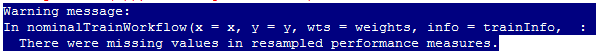#2017 iT 邦幫忙鐵人賽
DAY 27
1
Big Data

## Day27 R語言機器學習之類神經網路

• 輸入層(Input Layer)指的是輸入的X變數因子。
• 輸出層(OutputLayer)則是結果值Y的組合。
• 中間的隱藏層(Hidden Layer)則是負責運算的神經元，通常會以輸入層加上輸出層數目開根號決定單一層隱藏層中的神經元數目。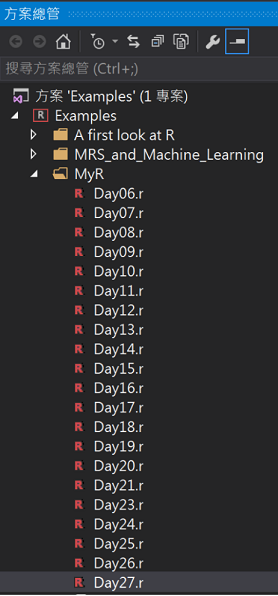## 單一隱藏層的feed-forward neural networks套件(nnet)

``````#(1)載入套件及資料
#從github下載套件 #plot.nnet
source_url('https://gist.githubusercontent.com/Peque/41a9e20d6687f2f3108d/raw/85e14f3a292e126f1454864427e3a189c2fe33f3/nnet_plot_update.r')
library(DMwR)
library(nnet)
library(reshape)
library(devtools)
library(scales)
library(ggplot2)
#範例使用irisdata
data(iris)

#(2)分為訓練組和測試組資料集
set.seed(1117)
#取得總筆數
n <- nrow(iris)
#設定訓練樣本數70%
t_size = round(0.7 * n)
#取出樣本數的idx
t_idx <- sample(seq_len(n), size = t_size)
#訓練組樣本
traindata <- iris[t_idx,]
#測試組樣本
testdata <- iris[ - t_idx,]

nnetM <- nnet(formula = Species ~ ., linout = T, size = 3, decay = 0.001, maxit = 1000, trace = T, data = traindata)

#(3)畫圖
plot.nnet(nnetM, wts.only = F)

#(4)預測
#test組執行預測
prediction <- predict(nnetM, testdata, type = 'class')

#預測結果
cm <- table(x = testdata\$Species, y = prediction, dnn = c("實際", "預測"))
cm
``````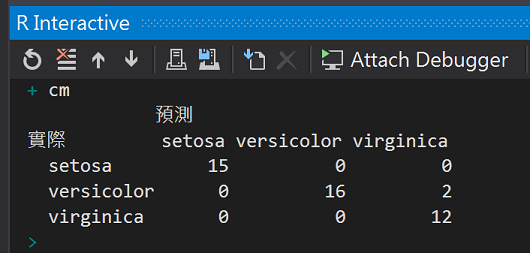``````nnetM <- nnet(formula = Species ~ ., linout = T, size = 6, decay = 0.001, maxit = 1000, trace = T, data = traindata)

plot.nnet(nnetM, wts.only = F)
``````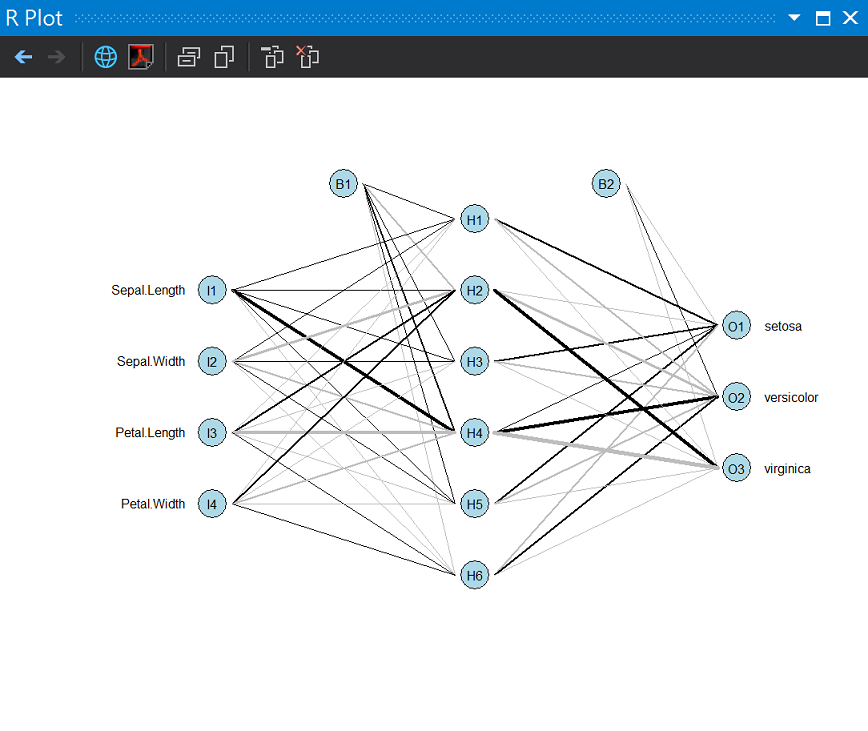## R語言中多個隱藏層的類神經網路套件neuralnet()

``````#載入套件
library("neuralnet")

#整理資料
data <- iris
data\$setosa <- ifelse(data\$Species == "setosa", 1, 0)
data\$versicolor <- ifelse(data\$Species == "versicolor", 1, 0)
data\$virginica <- ifelse(data\$Species == "virginica", 1, 0)

#訓練模型
f1 <- as.formula('setosa + versicolor + virginica  ~ Sepal.Length + Sepal.Width + Petal.Length + Petal.Width')
bpn <- neuralnet(formula = f1, data = data, hidden = c(2,4),learningrate = 0.01)
print(bpn)

#圖解BP
plot(bpn)
``````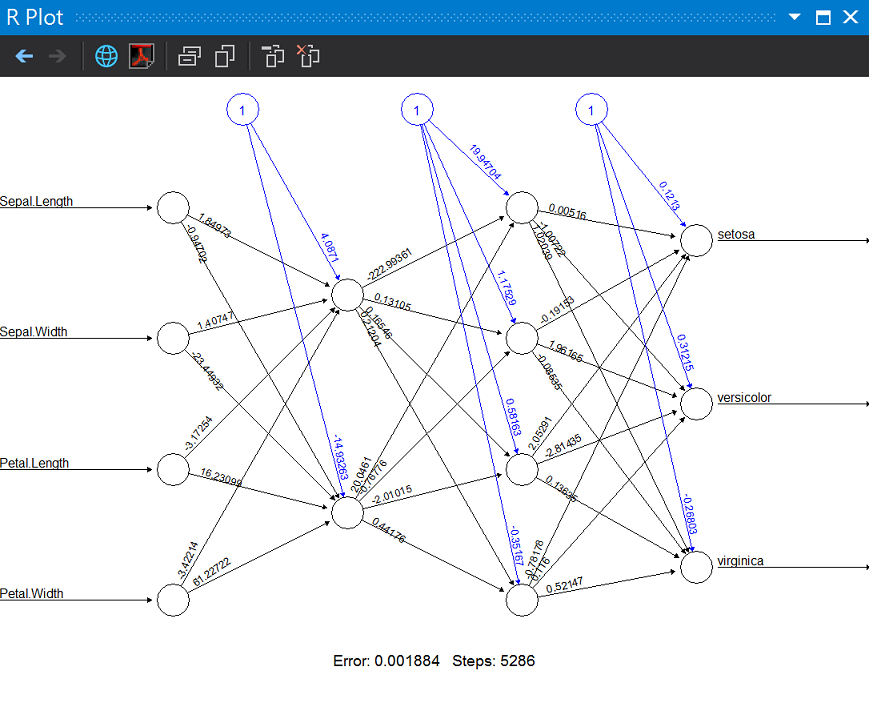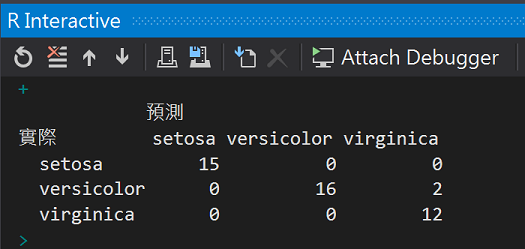## 調校參數Tuning Parameters

``````#調校參數
library(caret)

#預測最佳神經元參數組合
model <- train(form = f1, data = traindata, method = "neuralnet",
tuneGrid = expand.grid(.layer1 = c(1:4), .layer2 = c(1:4), .layer3 = c(0)), learningrate = 0.01)
model
``````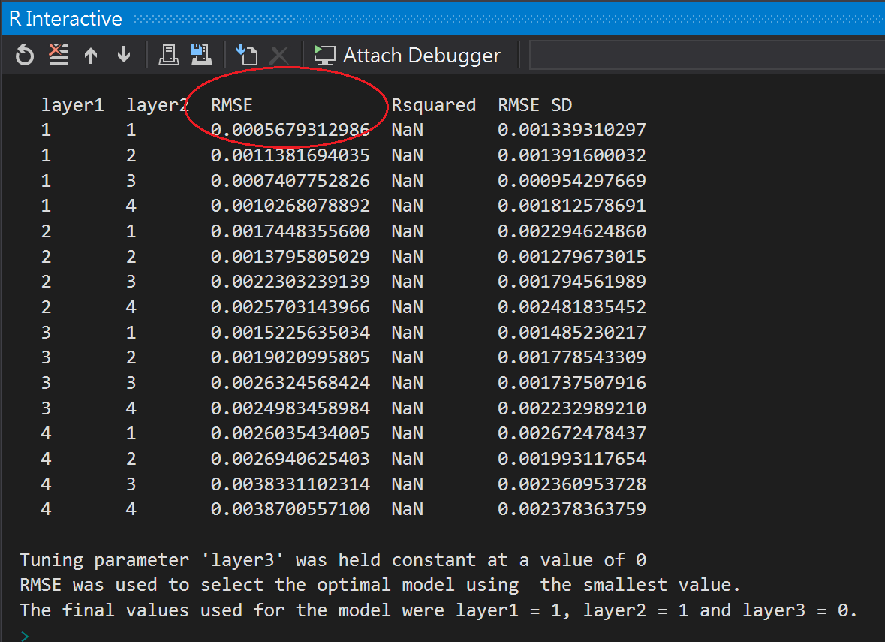「均方根誤差」(Root mean square error，RMSE) 是統計上的標準方法，用於查看不同資料集的比較方式，然後讓可以透過輸入刻度導入的差異變平滑。
RMSE 代表與實際值相比時，預測值的平均誤差。 它會以所有資料分割案例平均誤差的平方根，除以資料分割中的案例數目來計算，不包括目標屬性擁有遺漏值的資料列。

• neuralnet RSNNS
• 卷積神經網路(Convolutional neural network, CNN)
• 遞歸神經網路(Recurrent neural network)

## 參考:

nnet: Feed-Forward Neural Networks and Multinomial Log-Linear Models
https://cran.r-project.org/web/packages/nnet/index.html

Training of Neural Networks
https://cran.r-project.org/web/packages/neuralnet/index.html

https://msdn.microsoft.com/zh-tw/library/dn282375(v=sql.120).aspx2013-05攝於Salzburg,Austria

R語言與機器學習見面會30

### 1 則留言

0

Step1. 產生一組訓練資料
Step2. 使用neuralent函數
Step3. 產生此資料的類神經網路架構圖(模型)
Step4. 產生一組測試資料，使用caret套件，找出層中最適合的神經元數

A <- neuralnet(Sqrt~X1, sqrt.data, hidden=4, learningrate=0.01, threshold=0.01)

model <- train(form=A, sqrt.data, method = "neuralnet",
tuneGrid = expand.grid(.layer1 = c(1:4), .layer2 = c(0), .layer3=c(0)),
learningrate=0.01)